בתשובה לעוזי ו., 09/11/04 12:46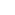הקובץ שצורף למכתב:260248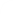עוזי ו. • בתשובה לעוזי ו. יום ג', 9/11/2004, 12:47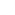First, there are only ten numbers that were measured in a wheel (the rest are additions of themselves). They created a field of their own when mathematical formulas were made during the process of observation by means of counting.

Every time you make formulas for a measurement a field is created for those measurements only. This is what happens to transcendental numbers, they create a field independent from the number’s field. They cannot be solved with the algebraic formulas that were created with the measurements made with the numbers we know as the only numbers. Numbers are physical lengths that create their own scale (have their own unit length) transcendental numbers (witch were measured or formulated somewhere else) cannot be compared with the scale that belongs to the numbers and have a short decimal expansion in the answer.

During division, we are comparing two lengths (numerator and denominator). When the unit length of both integers is equal, they will always meet (a mark they both reach at the same time when they are added) and the answer is always exact. When you compare two integers (numerator and denominator), with unit lengths unequal (they only reach the end at astronomical small unit lengths), the answer is what is called an infinite number.

An infinite number is just a regular division accompanied by a part of the small part that is left from the division in truncated form because the formula that was created for the numbers yields a small precision. The repeated part of a rational number repeats because it cannot be divided any more. The repetition goes forever because the formula that was created used a wheel that can count forever because the numbers return to their starting points repeatedly when counting (turning).

I figured out that if numbers greater than 10, are just additions of themselves, and I know that physical lengths have a physical limit of measurement (an atom for instance). That division p/q is a natural division plus the truncated part (according to me), I concluded: Irrational numbers have to be rational numbers with longer cycles of repeated truncated parts we cannot see with a division that shows up to 32 digits in our calculators.

While working on several conclusions I have made that confirm my thinking, I discovered several patrons.
I used the one patron I sent before (IRRATIONAL NUMBERS DO NOT EXIST file) to confirm the longer cycle irrational numbers have.

Last thing about transcendental numbers (non integers): they usually have been measured very precise, witch means their scale of precision (unit length) compared to the number’s is too great. The conclusion is that their cycle of the truncated part is extremely long but finite. How finite? Very large to the point it can easily reach the atom size. We still do not know how small a physical particle can reach. Another conclusion I have that is in the field of physics is that energy being a transformation of matter is in reality another form of matter and therefore physical too.

Check, the numbers and the cycle of the considered irrational number I used in the file IRRATIONAL NUMBERS DO NOT EXIST.
Its cycle repeats after 96 decimal places, for only an integer of only two digits (no decimal part). You can imagine the division for π, and several of its digits. It is simply too big even to see it with supercomputers.

One way to see π, would be to measure it again, create a formula that yields a small precision (one to three digits) and believe me, you would see its cycle.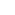מערכת האייל הקורא אינה אחראית לתוכן תגובות שנכתבו בידי קוראים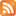RSS מאמרים | כתבו למערכת | אודות האתר | טרם התעדכנת | ארכיון | חיפוש | עזרה | תנאי שימוש © כל הזכויות שמורות